﻿ Lacunary Interpolation (0,2;3) Problem and Some Comparison from Quartic Splines

### Lacunary Interpolation (0,2;3) Problem and Some Comparison from Quartic Splines

Ambrish Kumar Pandey, Q S Ahmad, Kulbhushan SinghOPEN ACCESSPEER-REVIEWED

## Lacunary Interpolation (0,2;3) Problem and Some Comparison from Quartic Splines

Ambrish Kumar Pandey1, Q S Ahmad1,, Kulbhushan Singh2

1Department of Mathematics, Integral University, Lucknow

2Department of Mathematics and Computer Science , papua New Guinea University of Technology, Lae, Papuae New Guinea

### Abstract

This paper discuss a method for lacunary interpolation and their comparison with quartic splines in Generalised form as an local methods for solving lacunary interpolation problems using piecewise polynomials with certain specific properties.

• Pandey, Ambrish Kumar, Q S Ahmad, and Kulbhushan Singh. "Lacunary Interpolation (0,2;3) Problem and Some Comparison from Quartic Splines." American Journal of Applied Mathematics and Statistics 1.6 (2013): 117-120.
• Pandey, A. K. , Ahmad, Q. S. , & Singh, K. (2013). Lacunary Interpolation (0,2;3) Problem and Some Comparison from Quartic Splines. American Journal of Applied Mathematics and Statistics, 1(6), 117-120.
• Pandey, Ambrish Kumar, Q S Ahmad, and Kulbhushan Singh. "Lacunary Interpolation (0,2;3) Problem and Some Comparison from Quartic Splines." American Journal of Applied Mathematics and Statistics 1, no. 6 (2013): 117-120.

 Import into BibTeX Import into EndNote Import into RefMan Import into RefWorks

### 1. Introduction

During the last century, splines theory has received considerable attention. Lacunary interpolation was initiated in 1957 . Several researchers have studied the use of splines to solve such interpolation problems [2, 3, 4, 5]. All of these methods are global and require the solution of a large system of equations. The most appropriate method solving lacunary interpolation problems using piecewise polynomials with certain continuity properties.

Spline functions are a good tool for the numerical approximation of functions on the one hand and they also suggest new, challenging and rewarding problems on the other. Piecewise linear functions, as well as step functions, have been an important theoretical and practical tools for approximation of such functions. Lacunary interpolation by spline appears whenever observation gives scattered or irregular information about a function and it's derivatives. Also, the data in the problem of lacunary interpolation are values of the functions and of it's derivatives but without hermite condition in which consecutive derivatives are used at each nodes.

### 2. Construction

Let S(x) ε S(5)n,6 denote the class of sixtic splines S(x) on [0,1] such that

•  S(x) ε C5 [0, 1]

•  S(x) is a polynomial of degree six on each subinterval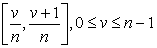(1)

It can be verified that if P(x) is a sixtic on [0, 1] then: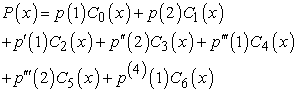Where: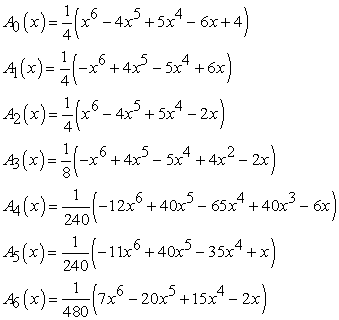(2)

For later references we have: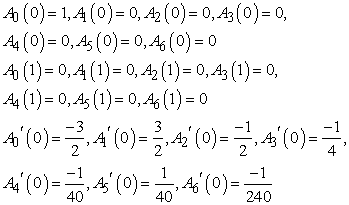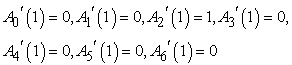and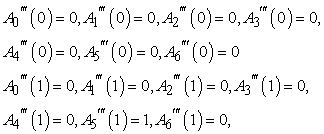and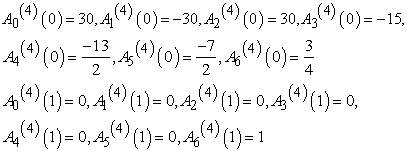and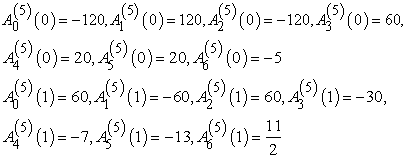Further, a sixtic P(x) on [1, 2] can be written as:Where: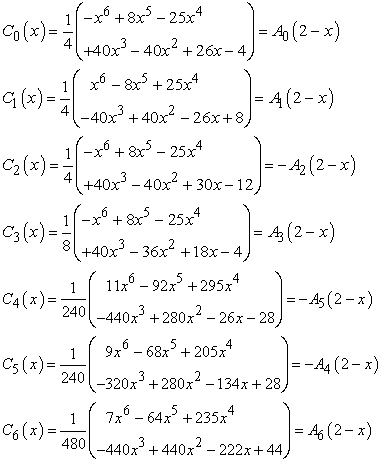(3)

It is easy to verify that a sixtic Q(x) on [0, 1] can be expressed in the following form: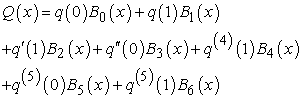(4)

Where: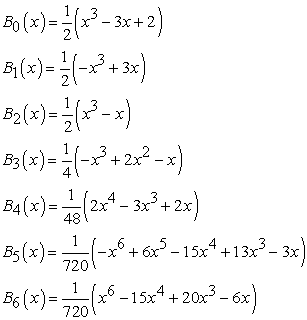(5)

for later references we have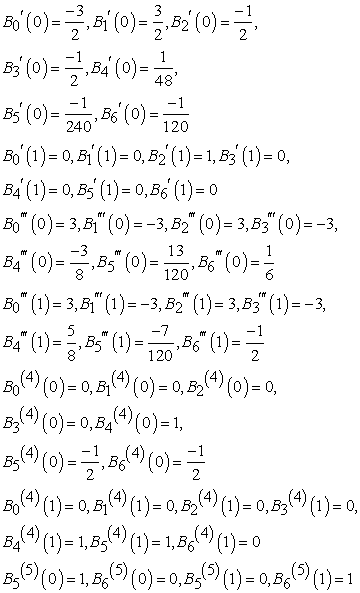Also a sixtic Q(x) on [1, 2] can be written as: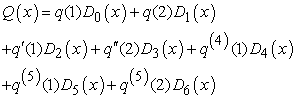(6)

Where: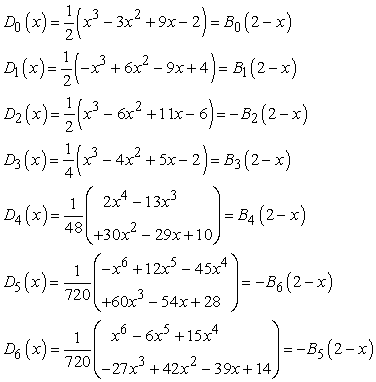(7)

### 3. The Approximation of the Spline Functions

Descriptions of the method: Let (Sn, C5) be the class of spline functions with respect to the set of knots xi. The spline functions will denoted by Si(x), where i = 0, 1,..., n. We shall prove the following:

Theorem 1 (Existence and Uniqueness)

For every odd integer n and for every set of 5n+9/2 real numbers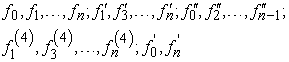there exists a unique S(x) ε S(5)n,6 such that: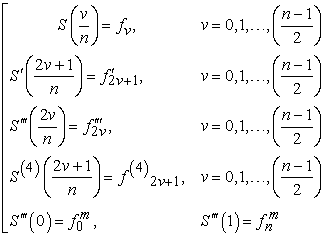Let fεC5[0,1] and n an odd integer. then the unique sixtic spline Sn(x) satisfying conditions of Theorem 3.1, with fv = f(v/n), v = 0,1,…,n;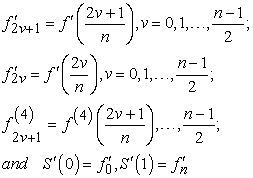(8)

we have: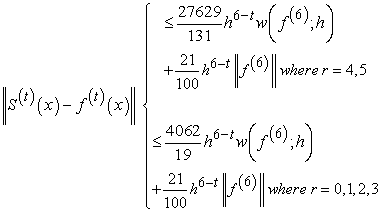Proof of Theorem 1

For a given S(x) ε S(5)n,6 set h = n-1, Mv = S(5) (vh+), v = 0,1,…..,n-1, Nv = S(5) (vh-), v = 0,1,….,n. Since, S(5) is linear in each internal (vh, (v+1)h), it is completely determined by the (2n) constants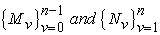. Also, if S(x) satisfies the requirements of Theorem 1 that for 2vh ≤ x ≤ (2v+1)h, v = 0,1,…, n-1/2 , it must have the following form: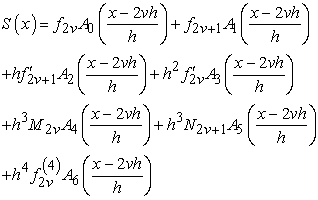(9)

and for (2v+1)h ≤ x ≤ (2v+2)h , v=0,1,…., n-3/2, S(x) has the form: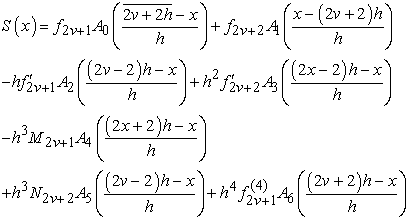(10)

We shall show that it is possible to determine the (2n) parameters, such that the function S(x) given by Eq. 9 and 10 will also satisfy (5) in Theorem 1 and S' (x), S"(x) and S(4) will be continuous on [0,1]. S(x) is continuous because of the interpolating condition Eq. 8 in Theorem 1, S'(x) and S(4)(x) are continuous on [0,1] except at the points (2vh) and (2v+1)h, respectively, v = 0, 1,… , n-1/2.

From Eq. 10 we see that Eq. 8 in Theorem 1 is equivalent to: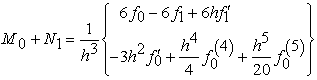(11)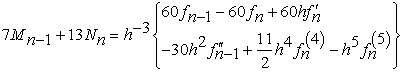(12)

Simple calculations show that S"((2v+2)h-) = S"((2v+2)h+) and S(5)((2v+2)h+) are equivalent to: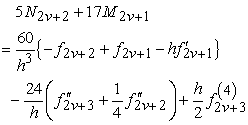(13)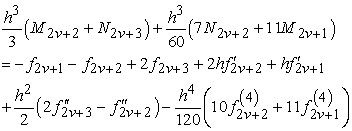(14)

Similarly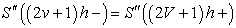and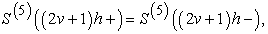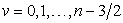are equivalent to: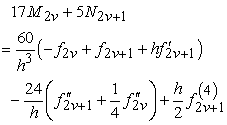(15)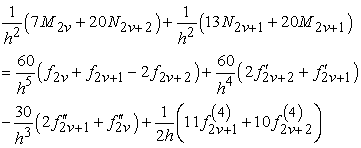(16)

Thus, the theorem will be established if we show that the system of linear Eq. 12-16 has a unique solution. This end will be achieved by showing that the homogeneous system corresponding to Eq. 12-16 has only zero solution.

The following is the homogeneous system of equations for v= 0,1,…, n-3/2: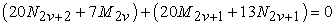(17)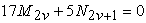(18)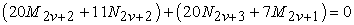(19)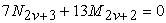(20)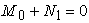(21)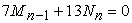(22)

Form Eq. 19 and 20 we have for v = 0, 1,…, n-3/2: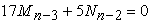and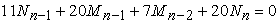(23)

Putting the values and Mn-1 = -13/7 Nn from Eq. 19 in 20 we have: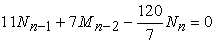(24)

Also, from Eq. 14 and 20 we have: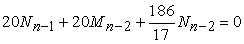(25)

and M0 = -N1 from Eq. 21.

Using Eq. 19 we obtain 17Mn-3+5Nn-2 and using Eq. 21 with 25 and 24 we have Mn-3 = Nn-2 = Mn-2 = Nn-1 = 0. Also obtain the system: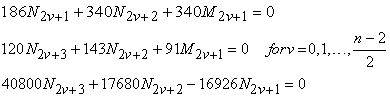By the same manner we get M0 = M1 = … = Mn-1 = 0 and N1=N2= N3 = … = Nn = 0, to solution of the homogeneous system for n = 4p and n = 4p+2.

### References

  J. BALAZS AND P. TURAN, Notes on interpolation. II, III, IV, Acta Math. Acud. Sci.Hungar, 8 (1957), 201-215; 9 (1958), 195-214, 243-258.In article  TH. FAWZY, Note on lacunary interpolation with splines. 1. (0, 3)-Lacunary interpolation,Ann. Univ. Sci. Budapest. Eiifciis Sect. Math. 28 (1985).In article  TH. FAWZY, Note on lacunary interpolation by splines. II, Ann. Univ. Sci. Budapest. Sect.Comput. 5, in press.In article  TH. FAWZY, Notes on lacunary interpolation by splines. III. (0, 2) Case, Acra Math. Acud.Sci. Hungar., in press.In article  TH. FAWZY, Lacunary interpolation by g-splines: (0, 2, 3) Case, Studia Sci. Math.Hungar., in press.In article